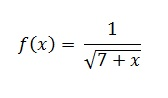# Linearization and Linear ApproximationLinearization, or linear approximation, is just one way of approximating a tangent line at a certain point.
Seeing as you need to take the derivative in order to get the tangent line, technically it’s an application of the derivative.

Like many tools (or arguably, all of them), linearization isn’t an exact science. It does, however, give you a very close approximation to the tangent line which will be adequate for most calculations. The slope equation can be written in many forms, including as an equation for the tangent line:
y – y0 = m(x – x0)y – f(x0).

## Linearization and Linear Approximation Example

Example Problem: Find the linearization of the following formula at x = 0:Step 1: Find the y-coordinate for the point. Plug the x-value into the formula:
y = f(0) = 1/√ 7 + 0
= 1/√ 7

Step 2: Plug your coordinates into the slope formula:
y – 1 / (√7) = m (x – 0)

Step 3: Take the derivative of the formula in Step 2:. Make the square root an exponent first:

• f(x) = (7 + x)1/2
• f′(x)= -½ (7 + x) – 3/2

Step 4: Insert the x-coordinate into the equation from Step 3 to get the slope:

• f′(0) = -1/(2(√73))
• y – 1 / √7) = -1/2(√73)(x – 0)

Step 5: Use algebra to simplify:

• y – 1 / √7 = -1 / 14√7 (x – 0)
• = (-1/14 √7)x + 1 / √7
• Factoring: = 1/√7 (1 – 1/14x)

That’s it!

Warning: Linear approximation is a good way to approximate values of f(x) as long as you stay close to x = a. However, the farther you get from x = a, the worse the approximation will be.CITE THIS AS:
Stephanie Glen. "Linearization and Linear Approximation" From StatisticsHowTo.com: Elementary Statistics for the rest of us! https://www.statisticshowto.com/calculus-problem-solving/linearization-linear-approximation/